Next: Rods and cables Up: Statics Previous: The principles of statics

## Equilibrium of a laminar object in a gravitational field

Consider a general laminar object which is free to pivot about a fixed perpendicular axis. Assuming that the object is placed in a uniform gravitational field (such as that on the surface of the Earth), what is the object's equilibrium configuration in this field?

Letrepresent the pivot point, and let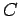be the centre of mass of the object. See Fig. 90. Suppose thatrepresents the distance between pointsand, whereasis the angle subtended between the line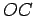and the downward vertical. There are two external forces acting on the object. First, there is the downward force,, due to gravity, which acts at the centre of mass. Second, there is the reaction,, due to the pivot, which acts at the pivot point. Here,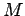is the mass of the object, andis the acceleration due to gravity.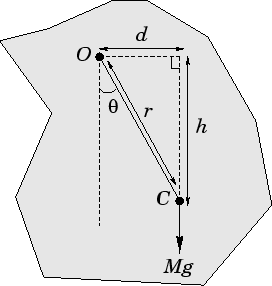Two conditions must be satisfied in order for a given configuration of the object shown in Fig. 90 to represent an equilibrium configuration. First, there must be zero net external force acting on the object. This implies that the reaction,, is equal and opposite to the gravitational force,. In other words, the reaction is of magnitudeand is directed vertically upwards. The second condition is that there must be zero net torque acting about the pivot point. Now, the reaction,, does not generate a torque, since it acts at the pivot point. Moreover, the torque associated with the gravitational force,, is simply the magnitude of this force times the length of the lever arm,(see Fig. 90). Hence, the net torque acting on the system about the pivot point is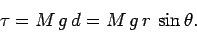(468)

Setting this torque to zero, we obtain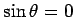, which implies that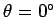. In other words, the equilibrium configuration of a general laminar object (which is free to rotate about a fixed perpendicular axis in a uniform gravitational field) is that in which the centre of mass of the object is aligned vertically below the pivot point.

Incidentally, we can use the above result to experimentally determine the centre of mass of a given laminar object. We would need to suspend the object from two different pivot points, successively. In each equilibrium configuration, we would mark a line running vertically downward from the pivot point, using a plumb-line. The crossing point of these two lines would indicate the position of the centre of mass.

Our discussion of the equilibrium configuration of the laminar object shown in Fig. 90 is not quite complete. We have determined that the condition which must be satisfied by an equilibrium state is. However, there are, in fact, two physical roots of this equation. The first,, corresponds to the case where the centre of mass of the object is aligned vertically below the pivot point. The second,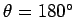, corresponds to the case where the centre of mass is aligned vertically above the pivot point. Of course, the former root is far more important than the latter, since the former root corresponds to a stable equilibrium, whereas the latter corresponds to an unstable equilibrium. We recall, from Sect. 5.7, that when a system is slightly disturbed from a stable equilibrium then the forces and torques which act upon it tend to return it to this equilibrium, and vice versa for an unstable equilibrium. The easiest way to distinguish between stable and unstable equilibria, in the present case, is to evaluate the gravitational potential energy of the system. The potential energy of the object shown in Fig. 90, calculated using the height of the pivot as the reference height, is simply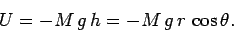(469)

(Note that the gravitational potential energy of an extended object can be calculated by imagining that all of the mass of the object is concentrated at its centre of mass.) It can be seen thatcorresponds to a minimum of this potential, whereascorresponds to a maximum. This is in accordance with Sect. 5.7, where it was demonstrated that whenever an object moves in a conservative force-field (such as a gravitational field), the stable equilibrium points correspond to minima of the potential energy associated with this field, whereas the unstable equilibrium points correspond to maxima.Next: Rods and cables Up: Statics Previous: The principles of statics
Richard Fitzpatrick 2006-02-02